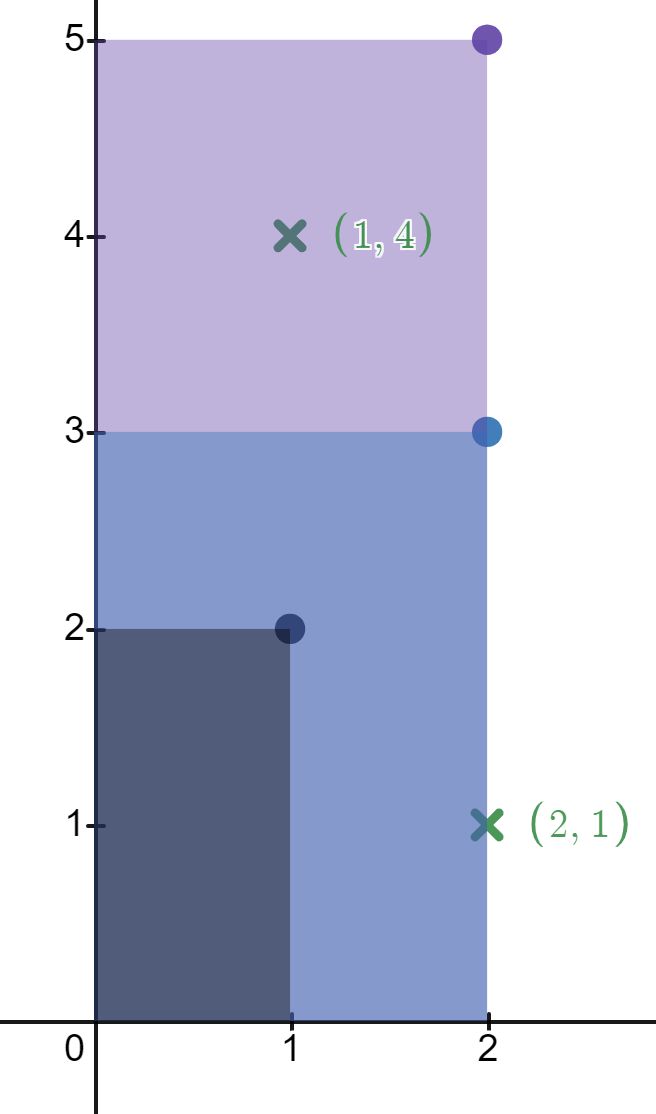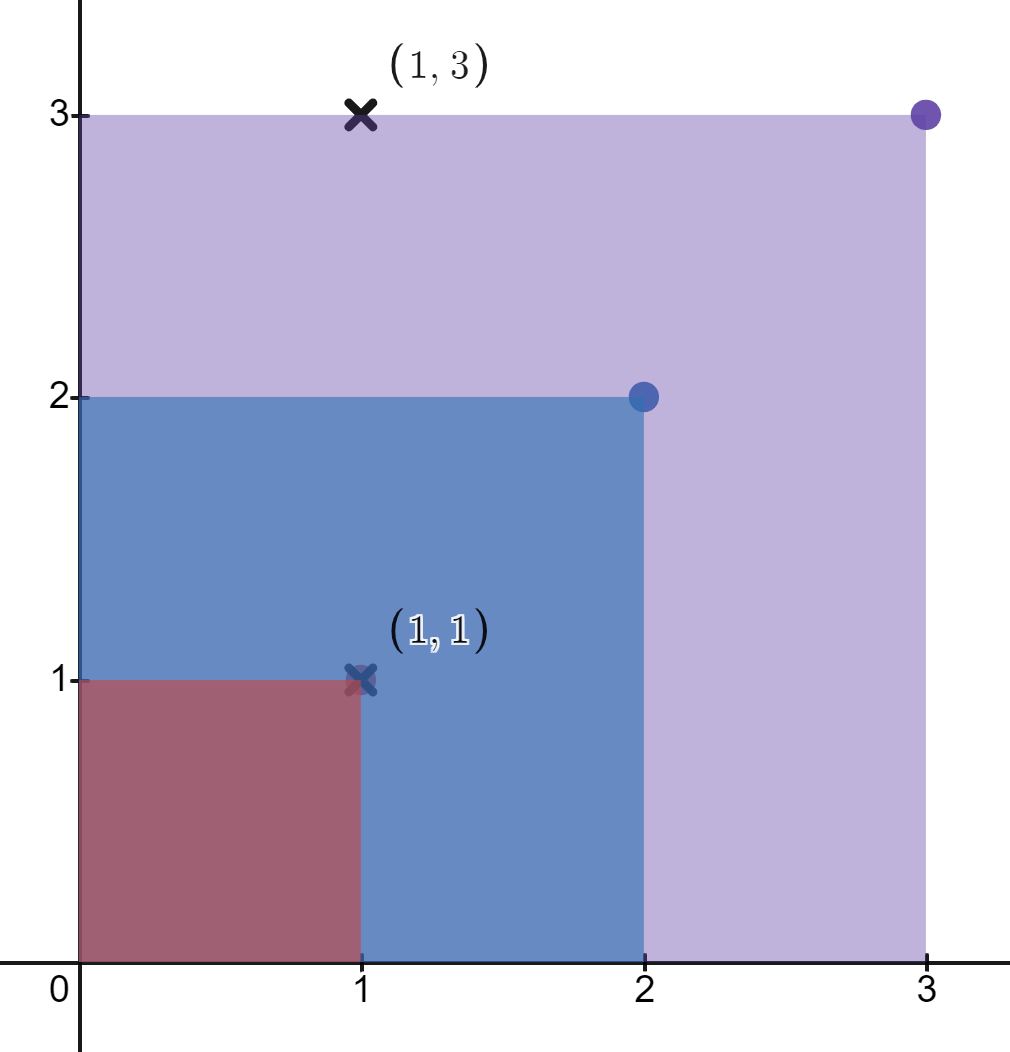# Count Number of Rectangles Containing Each Point Solution Leetcode

## Count Number of Rectangles Containing Each Point Solution Leetcode

You are given a 2D integer array `rectangles` where `rectangles[i] = [li, hi]` indicates that `ith` rectangle has a length of `li` and a height of `hi`. You are also given a 2D integer array `points` where `points[j] = [xj, yj]` is a point with coordinates `(xj, yj)`.

The `ith` rectangle has its bottom-left corner point at the coordinates `(0, 0)` and its top-right corner point at `(li, hi)`.

Return an integer array `count` of length `points.length` where `count[j]` is the number of rectangles that contain the `jth` point.

The `ith` rectangle contains the `jth` point if `0 <= xj <= li` and `0 <= yj <= hi`. Note that points that lie on the edges of a rectangle are also considered to be contained by that rectangle.

## Example 1: Count Number of Rectangles Containing Each Point Solution Leetcode```Input: rectangles = [[1,2],[2,3],[2,5]], points = [[2,1],[1,4]]
Output: [2,1]
Explanation:
The first rectangle contains no points.
The second rectangle contains only the point (2, 1).
The third rectangle contains the points (2, 1) and (1, 4).
The number of rectangles that contain the point (2, 1) is 2.
The number of rectangles that contain the point (1, 4) is 1.
Therefore, we return [2, 1].
```

## Example 2: Count Number of Rectangles Containing Each Point Solution Leetcode```Input: rectangles = [[1,1],[2,2],[3,3]], points = [[1,3],[1,1]]
Output: [1,3]
Explanation:
The first rectangle contains only the point (1, 1).
The second rectangle contains only the point (1, 1).
The third rectangle contains the points (1, 3) and (1, 1).
The number of rectangles that contain the point (1, 3) is 1.
The number of rectangles that contain the point (1, 1) is 3.
Therefore, we return [1, 3].
```

## Constraints: Count Number of Rectangles Containing Each Point Solution Leetcode

• `1 <= rectangles.length, points.length <= 5 * 104`
• `rectangles[i].length == points[j].length == 2`
• `1 <= li, xj <= 109`
• `1 <= hi, yj <= 100`
• All the `rectangles` are unique.
• All the `points` are unique.